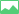# Distribution of Ions In Resting Axons

Last update:

The ion concentrations in the extracellular fluid (ECF) and intracellular fluid (ICF) of a resting axon can bemeasured.

1
2

These concentrations are often expressed in millimolar (mM) units.

## Molarity:• Molarity (M) is the number of moles of a solute in one liter of solution.
• One Mole of a solute contains 6.023 x 10^23 particles.
• Thus, a 1 molar (M) solution contains 6.023 x 10^23 particles in a liter of solution.
• A 1 millimolar (mM) solution is 1000 X less concentrated than a 1 M solution.

Phew, lots of new information to take in. Why not try testing yourself with the technique of active recall?

1
2
3
1
2
3